Calculate Area between Limiting Angles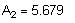Check if this condition meets minimum area requirements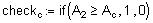Check Maximum Righting Arm Locations, per 46 CFR 174.145(d)
The Maximum Righting Arm shall occur at an angle of heel of at least 25 degrees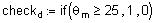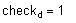Sheet 2 of 3
Check Angle of Vanishing Stability, as per 46 CFR 174.145(e)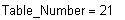The Angle of Vanishing Stability is first calculated and then checked to see that it is greater than 60 degrees.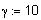Iteration Fineness, fractions of a degree
Initial Angle of Range needed for iterations below, degrees.Iteration Values, first value of range important because of multiple intercepts possible for some cases, degrees.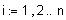Tolerance Allowed on GZ Difference, meters
The Maximum Available Righting Arm in this region is: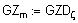Angle Corresponding to this Maximum Righting Arm, degrees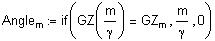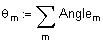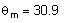Determine Limiting Angle, as per 46 CFR 174.145(b)
Limiting Angle, the least of 40 degrees, Angle of Maximum Righting Arm and Downflooding Angle.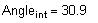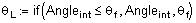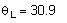Check Area from Zero Degrees to Limiting Angle, as per 46 CFR 174.145(b)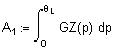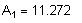Calculate Area up to Limiting Angle
Check if this condition meets minimum area requirements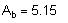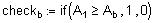Check Area from 30 Degrees to Limiting Angle, as per 46 CFR 174.145(c)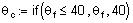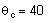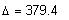Displacement, metric tonsArea Available from 30 deg. to Limiting Angle, meters·deg.Keel to Metacenter, meters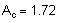Area Required from 30 deg. to Limiting Angle, meters·deg.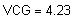KG value evaluated, metersAngle at Maximum Righting Arm, deg.
Summary of Calculated Results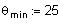Minimum Angle for Maximum Righting Arm, deg.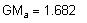Metacentric Height Available, meters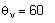Intercept Angle, End of Positive Stability Range, degreesResults of this analysis, satisfactory only if equal to one.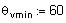Min. Intercept Angle for Positive Stability Range, degrees
Sheet 3 of 3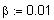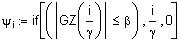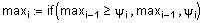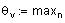Intercept Angle
The effectiveness of this value meeting the requirements is checked next: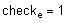Summary of Results
This value is one if vessel meets the requirements of proposed 46 CFR 174.145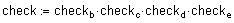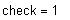Graph of Righting Arm Curve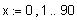Curve of Statical Stability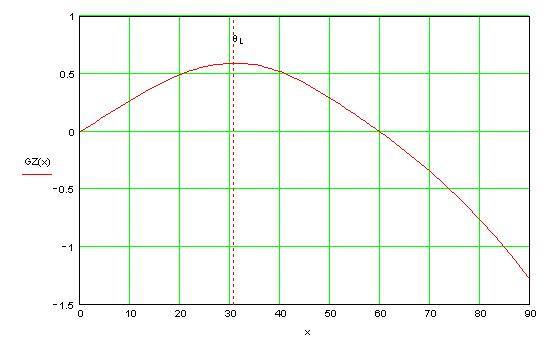Summary of Criterion Requirements
Summary of Important InputsArea Available to Limiting Angle, meters·deg.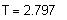Draft, metersArea Required to Limiting Angle, meters·deg.
*
26.5m x 9.5m x 4.3m
*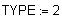Filename of the calculations that are contained here.
*
TBL_F2A.MCD
Vessel Draft, meters. When INPUT =1 put in the mean draft for the condition under evaluation. When INPUT=2 it usually means the level draft is being evaluated. Otherwise it is the LCF draft.
Vertical Center of Gravity, meters, above Baseline. The is the same VCG (or KG) value that was used for constructing the "Cross Curves of Stability." This value facilitates fast cross checking with the other vessel data.
*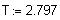*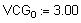Downflooding Angle, based on the above draft and the vessel's geometry. Requires the lowest location where the hull begins to flood after all WT openings have been closed for bad weather.
*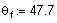Condition Inputs from Hydrostatics Program or Curves of Form & Cross Curves of Stability
Input Righting Arms are below. No free surface effects are included in this data .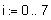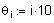*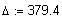Vessel Displacement, Metric Tons.
Keel to Metacenter for the Transverse Direction, meters, this value obtained at the same time as the above displacement for the level trim case. Where KMT = VCB + BMT.
Tow Vessel, Dynamic Weather Criterion, VCG Check, as per 46 CFR 174.145
Table F2A
*
Date: 12 July 2004
This analysis is based on the dynamic weather requirements contained in CFR Title 46 Stability, Subpart E -Special Rules Pertaining to Tugboats and Towboats, 174.140 and 174.145 . This analysis was prepared in a Windows 95 operating system (or later version) with MathCAD version 6 (or later version).
*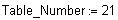*
Units: Metric
Vertical Center of Gravity, meters, above the Baseline is positive. This value should already include the free surface correction.
Vessel Inputs
*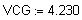*
Tug Company Name
Client
*
M/T Tugboat Name
Vessel Name
TYPE =1 when a specific VCG is being checked. TYPE=2 when seeking the maximum allowable value for this criterion. In a TYPE 2 analysis this VCG value is ITERATED to obtain the highest possible value that meets all this criterion's requirements.
*
Z-Drive Tug
Vessel Type
Vessel Particulars,
LBP x Beam x Depth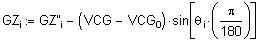This last column of table below shows Corrected Righting Arms for VCG value.
The formula for representing GZ as a function of theda is derived as follows: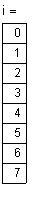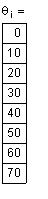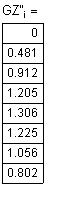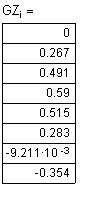A cubic spline is used to represent the end conditions.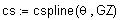The above function is redefined below in terms of a cubic interpolation function.This function is graphed on the last page of this
analysis.
Determine Angle of Maximum Righting Arm, as per 46 CFR 174.145(b)(2)
Determining Maximum Righting Arm & its Corresponding Angle, Range from Zero to 90 Degrees
The Angle corresponding to maximum righting arm is obtained from the following iterative process.
This is the initialization value, meters.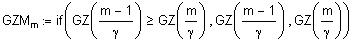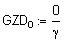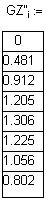*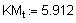Longitudinal Center of Gravity, meters, aft of Midships is positive. For the level trim case this equals the LCB value and it is obtained at the same time as the displacement above.
*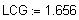If Input = 1 data for this condition is directly from a hydrostatics program, if Input =2 data are from the "Curves of Form" and the "Cross Curves of Stability."
*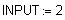Vessel Inputs for Tow Vessel as Defined by 46 CFR 174.145
Minimum Moment Area Required from zero degrees to critical angle, in metric units of meter·degrees
Minimum Moment Area Required between 30 degrees and crtical angle, in metric units of meter·degrees
*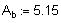*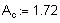Metacentric Height Calculations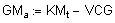Available Metacentric Height, meters
Sheet 1 of 3
Calculations for Righting Arm CurveIn this formula a correction VCG 0 is made to the Righting Arm Curve.
The procedures, techniques and presentation contained on these pages are copyrighted. Only purchasers of the template may utilize them, any other use is strictly prohibited.